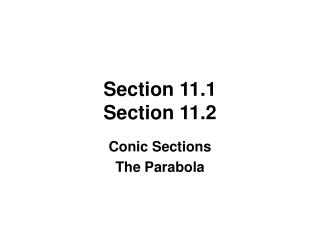DownloadDownload PresentationSection 11.1 Section 11.2

# Section 11.1 Section 11.2

Télécharger la présentation## Section 11.1 Section 11.2

- - - - - - - - - - - - - - - - - - - - - - - - - - - E N D - - - - - - - - - - - - - - - - - - - - - - - - - - -
##### Presentation Transcript

1. Section 11.1Section 11.2 Conic Sections The Parabola

2. THE CONIC SECTIONS The four conic sections are formed by the four ways that a plane can intersect a cone.

3. The standard form of an equation of a circle with center (h, k) and radius r is CIRCLES A circle is the set of points that are equidistant from a fixed point (the center). This distance is called the radius.

4. PARABOLAS A parabola is the set of points in the plane that are equidistant from a fixed line (the directrix) and a fixed point (the focus) not on the directrix. a

5. PARABOLAS WITH VERTEXAT THE ORIGIN Using algebra, we can show that the standard form of the equation of a parabola whose vertex is at the origin is x2 = 4ay if the axis of symmetry is vertical. y2 = 4ax if the axis of symmetry is horizontal. NOTE: In both cases the focus is |a| units “inside” the parabola; the directrix is |a| units “outside.”

6. PARABOLAS WITHVERTEX AT (h, k) Using translations as discussed in Section 3.5, we can find the standard form of an equation for a parabola whose vertex is at (h, k). if the axis of symmetry is vertical. if the axis of symmetry is horizontal.